# Hyperbolic partial differential equation

(diff) ← Older revision | Latest revision (diff) | Newer revision → (diff)

at a given point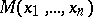A partial differential equation for which the Cauchy problem is uniquely solvable for initial data specified in a neighbourhood ofon any non-characteristic surface (cf. Characteristic surface). In particular, a partial differential equation for which the normal cone has no imaginary zones is a hyperbolic partial differential equation. The differential equation(*)

where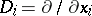(),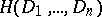is a homogeneous polynomial of degree, while the polynomialis of lower degree than, is a hyperbolic partial differential equation if its characteristic equation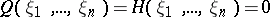hasdifferent real solutions with respect to one of the variables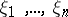, the remaining ones being fixed. Any equation (*) of the first order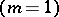with real coefficients is a hyperbolic partial differential equation. A second-order equationis hyperbolic if the quadratic formis positive definite.

The special variable among thesuch that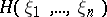hasdifferent real solutions for each set of fixed values of the other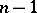is often taken to be(time). One speaks then of a (strictly) hyperbolic equation or an equation of (strictly) hyperbolic type with respect to the-direction. More generally one considers hyperbolicity with respect to a vector[a1].

A polynomialof degreewith principal partis called hyperbolic with respect to the real vectorif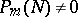and there exists a number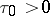such thatIfis such thatand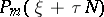has only simple real roots for every real, thenis said to be strictly hyperbolic or hyperbolic in the sense of Petrovskii.

The Cauchy problem for a constant-coefficient differential operatorwith data on a non-characteristic plane is well posed for arbitrary lower-order terms if and only ifis strictly hyperbolic. For a discussion of similar matters for polynomialswith variable coefficients cf. [a2].

For a system of higher-order linear partial differential equations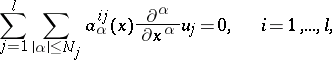where, is a hyperbolic system of partial differential equations in the sense of Petrovskii if the determinant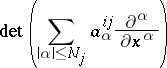calculated in the ring of differential operators is a hyperbolic polynomial in the sense of Petrovskii (as a polynomial of degree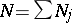). The Cauchy problem for a system that is hyperbolic in this sense is well posed [a3], [a4].

Instead of strictly hyperbolic one also finds the term strongly hyperbolic and instead of hyperbolic also weakly hyperbolic (which is therefore the case in which the lower-order terms ofdo matter).

How to Cite This Entry:
Hyperbolic partial differential equation. Encyclopedia of Mathematics. URL: http://encyclopediaofmath.org/index.php?title=Hyperbolic_partial_differential_equation&oldid=16785
This article was adapted from an original article by B.L. Rozhdestvenskii (originator), which appeared in Encyclopedia of Mathematics - ISBN 1402006098. See original article